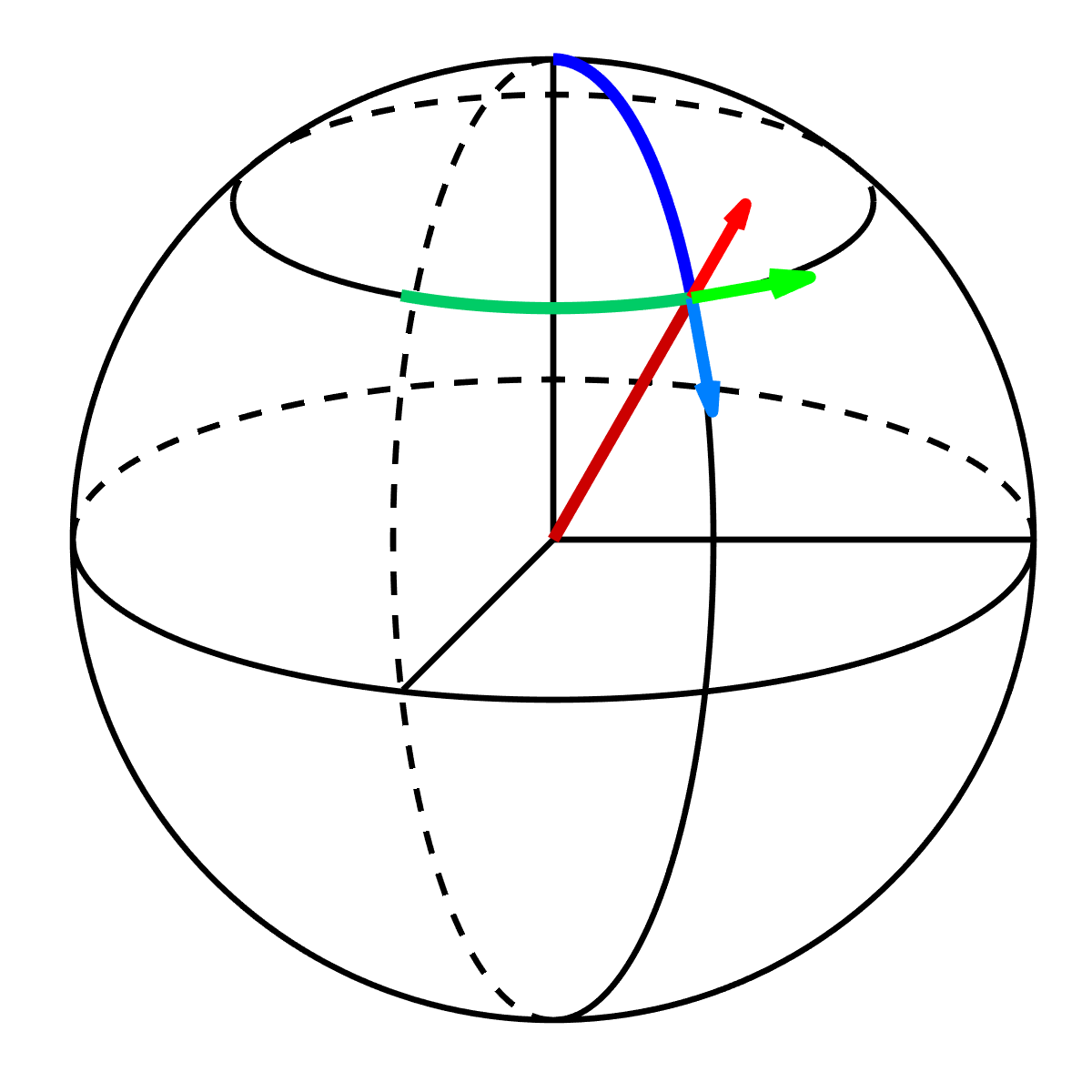Coursera# [100% Off] Vector Calculus for Engineers Free Course Coupon

Expires:

To Enroll Free in this course, Click on Audit.

28.0 hr
English
0\$ 45.00\$
Get the coupon in the end of description.

### Description

Vector Calculus for Engineers covers both basic theory and applications. In the first week we learn about scalar and vector fields, in the second week about differentiating fields, in the third week about multidimensional integration and curvilinear coordinate systems. The fourth week covers line and surface integrals, and the fifth week covers the fundamental theorems of vector calculus, including the gradient theorem, the divergence theorem and Stokes’ theorem. These theorems are needed in core engineering subjects such as Electromagnetism and Fluid Mechanics. Instead of Vector Calculus, some universities might call this course Multivariable or Multivariate Calculus or Calculus 3. Two semesters of single variable calculus (differentiation and integration) are a prerequisite. The course is organized into 53 short lecture videos, with a few problems to solve following each video. And after each substantial topic, there is a short practice quiz. Solutions to the problems and practice quizzes can be found in instructor-provided lecture notes. There are a total of five weeks to the course, and at the end of each week there is an assessed quiz.
If the coupon is not opening, disable Adblock, or try another browser.